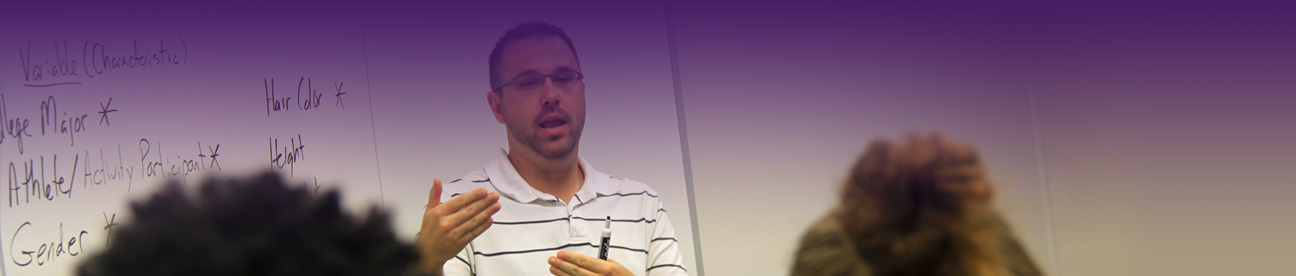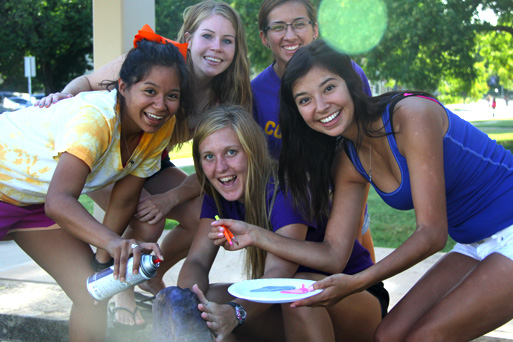# BS Major in Mathematics

### B.S., Major in Mathematics

#### Major requirements*

• MATH215 Introduction to Statistics and Probability (3 hours)
• MATH222 Calculus 1 (5 hours)
• MATH223 Calculus 2 (5 hours)
• MATH224 Calculus 3 (5 hours)
• MATH319 Linear Algebra (3 hours)
• MATH320 Introduction to Advanced Mathematics (3 hours)
• MATH321 Ordinary Differential Equations (3 hours)
• MATH331 Foundations of Geometry (3 hours)
• MATH444 Abstract Algebra (3 hours)
• MATH445 Mathematical Analysis (3 hours)
• MATH447 Complex Variables (3 hours)

#### Cognate requirements

• CPTR112 Programming 1 (or any other high-level programming language) (Minimum 3 hours)
• PHYS211 General Physics 1 (5 hours)
• PHYS212 General Physics 2 (5 hours)